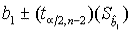### CFA Practice Question

There are 985 practice questions for this topic.

### CFA Practice Question

Which of the following statements is (are) true with respect to the regression coefficient?

I. As the sample standard error of the regression coefficient decreases, the range of possible values for the regression coefficient of the population increases.
II. The appropriate test statistic for analyzing sample regression coefficient is the t-test.
III. If zero is a value within the confidence interval of the regression coefficient, then it implies that there is no relation between the dependent and independent variable.
IV. The regression coefficient may only take on values between -1 and +1.
A. II
B. I, II and IV
C. II and III
Explanation: I is incorrect because as the sample standard error of the regression coefficient decreases, the implication is that the sample regression coefficient is an even more accurate representation of the population regression coefficient. Therefore, the range of possible values for the regression coefficient of the population around the sample estimate, decreases. The confidence interval for a regression coefficient may be found as follows:III is not necessarily true. If zero is a value within the confidence interval of the regression coefficient, then it simply implies that "it is possible" that there is no relation between the dependent and independent variable.

IV is incorrect because the regression coefficient may only take on any possible values between negative infinity and positive infinity. It's correlation coefficient that is restrained between -1 and +1.